﻿ 水下航行器动力电池组设计与性能研究
 舰船科学技术2020, Vol. 42Issue (6): 80-84    DOI: 10.3404/j.issn.1672-7649.2020.06.016PDF

1. 中国船舶重工集团公司第七一三研究所，河南 郑州 450015;
2. 河南省水下智能装备重点实验室，河南 郑州 450015;
3. 中国人民解放军92578部队，北京 100161

Research on design and performance of underwater vehicile power battery pack
LI Guang-hua1,2, WANG Xi-na3, LU Bing-ju1,2, DONG Ping1,2, GONG Shao-feng1,2
1. The 713 Research Institute of CSIC, Zhengzhou 450015, China;
2. Henan Key Laboratory of Underwater intelligent Equipment, Zhengzhou 450015, China;
3. No. 92578 Unit of PLA, Beijing 100161, China
Abstract: In this paper, the design ideas and solutions for a certain type of underwater vehicle power battery pack were proposed.For actual ambient temperature and closed space cooling conditions, by the method of theoretical analysis、experimental testing and simulation calculation, the effect of ambient temperature on the discharge characteristics and temperature rise characteristics of power battery pack was investigated.The research results can provide a basis for the accurate evaluation of the working stability, thermal safety and life time of the power battery pack.
Key words: power battery pack     discharge characteristics     temperature rise characteristics     simulation calculation
0 引　言

1 水下航行器动力电池组方案设计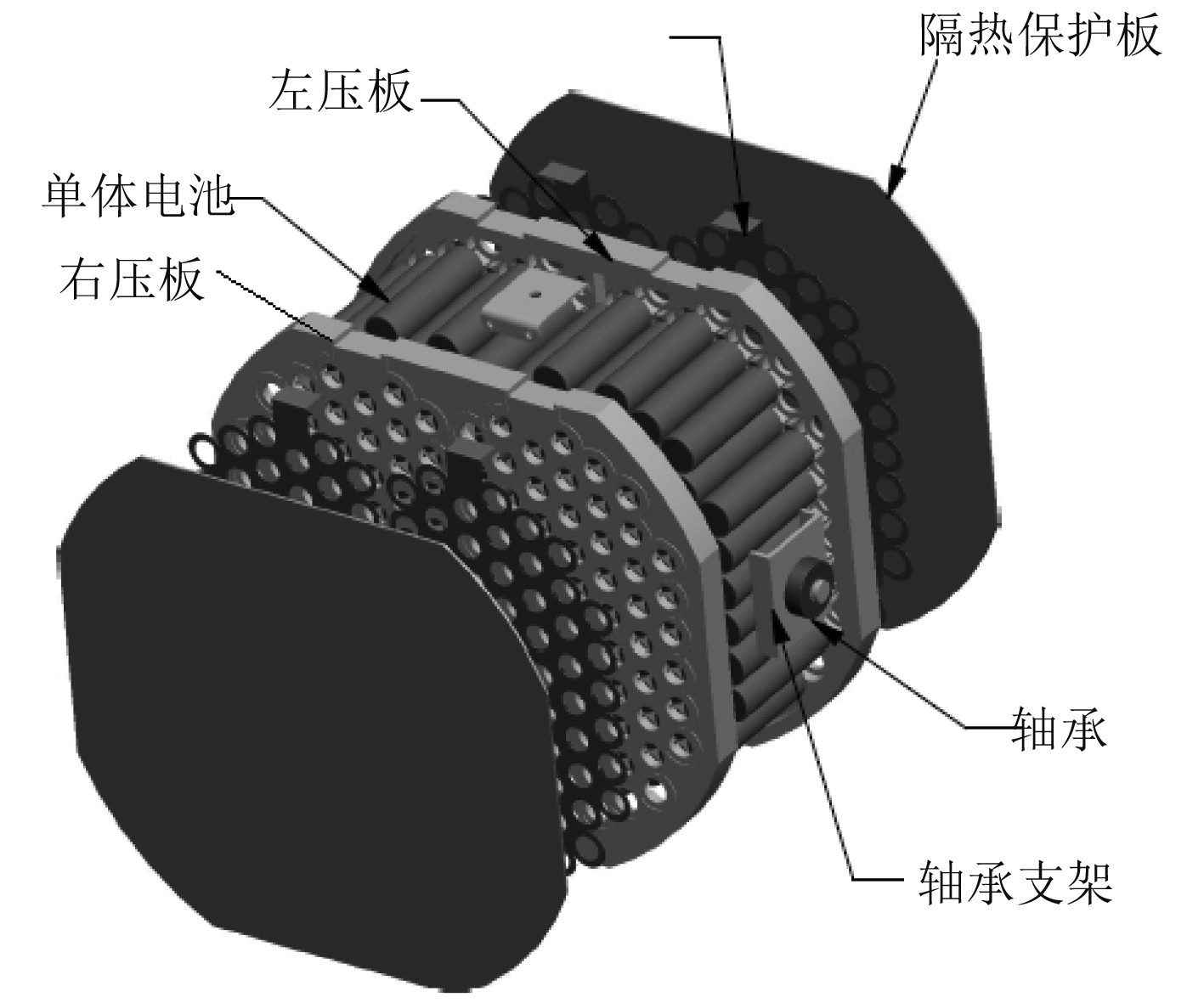图 1 某型动力电池组结构组成 Fig. 1 Structural composition of power battery pack
2 数学物理模型 2.1 圆柱形锂电池传热模型

 $\rho {{{C}}_p}\frac{{\partial T}}{{\partial {{t}}}} = {\lambda _r}\left( {\frac{{{\partial ^2}T}}{{\partial {r^2}}} + \frac{{\partial T}}{{r\partial r}}} \right) + {\lambda _\theta }\frac{1}{{{r^2}}}\frac{{{\partial ^2}T}}{{\partial {\theta ^2}}} + {\lambda _Z}\frac{1}{{{r^2}}}\frac{{{\partial ^2}T}}{{\partial {Z^2}}} + {q^*}\text{。}$

 ${{Q = (}}\sum\limits_{i = 1}^m {{{{h}}_i}} {A_i}\Delta {T_i})\Delta t\text{。}$

 $G{{{r}}_\delta } = \frac{{g\alpha \Delta t{\delta ^3}}}{{{\nu ^2}}}\text{。}$

 ${{h}} = \frac{\lambda }{\delta }\text{。}$
2.2 生热模型

 ${{q}} = \frac{I}{V}\left[ {\left( {E - U} \right) - T\frac{{{\rm d}E}}{{{\rm d}T}}} \right]\text{。}$

 ${{q}} = \frac{{{I^2}{{{R}}_{{{total}}}}}}{V}\text{。}$

3 试验测试

3.1 试验过程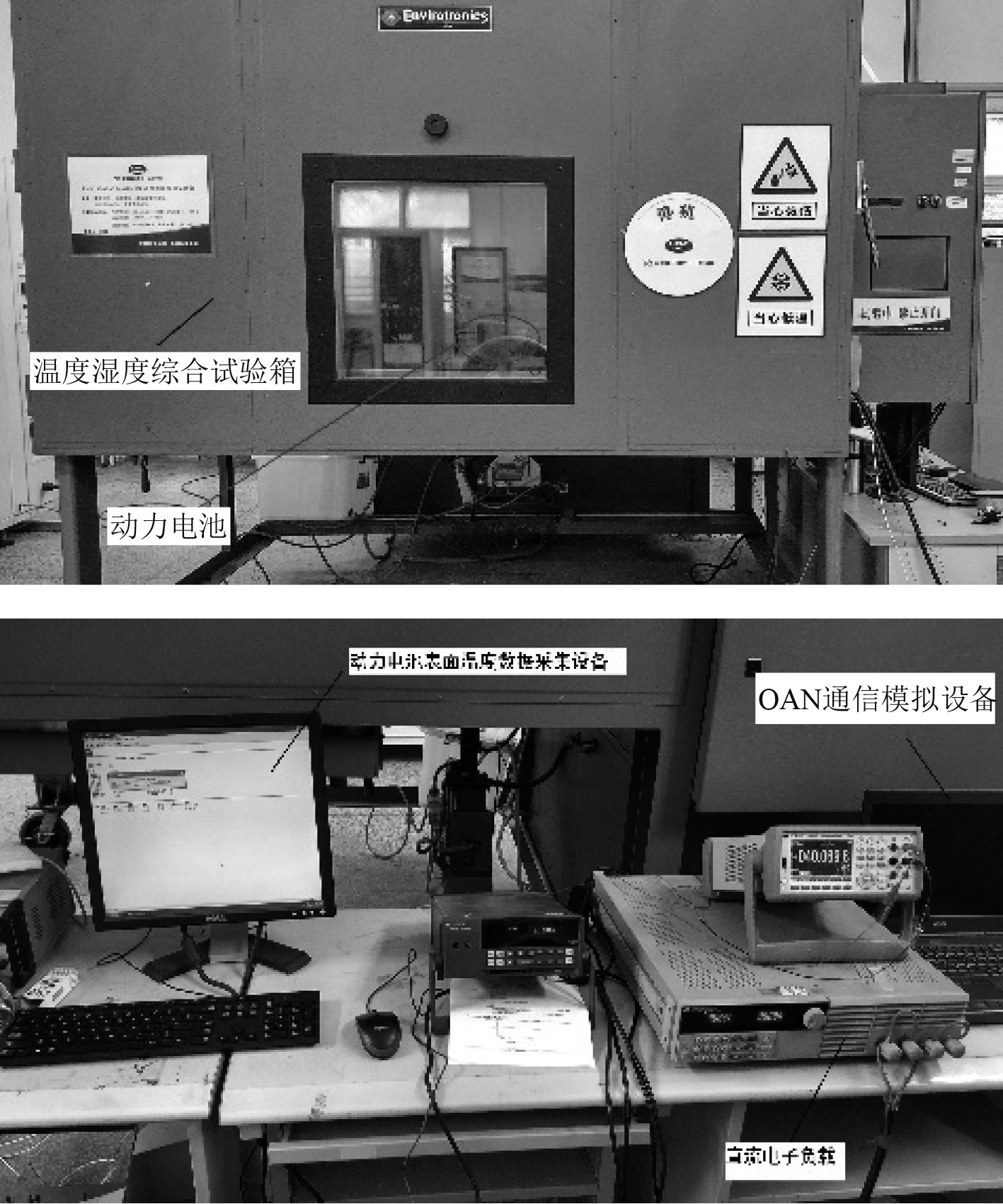图 2 动力电池组试验装置 Fig. 2 Power Battery Pack Test Device

3.2 试验结果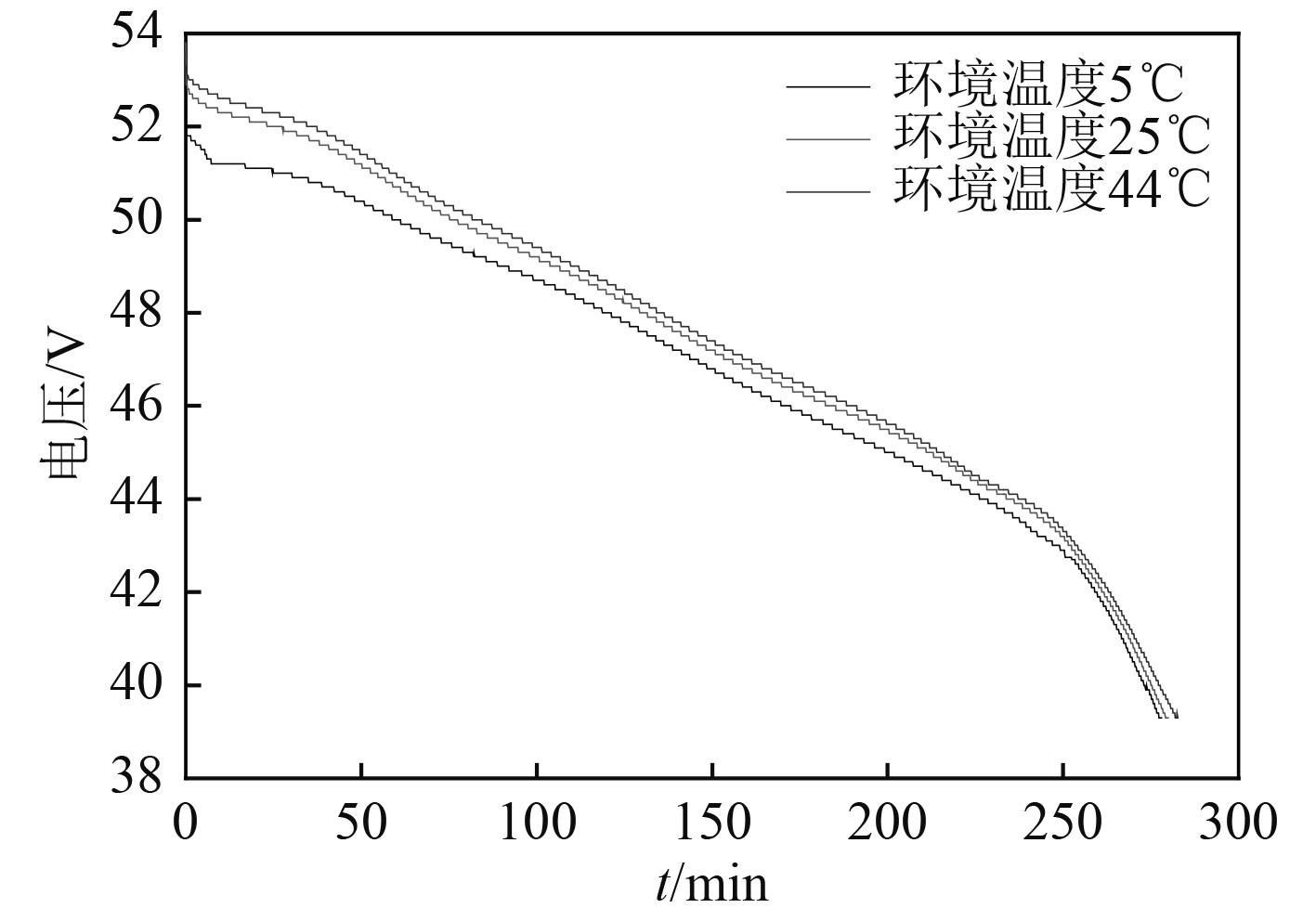图 3 不同温度下的放电电压曲线 Fig. 3 Discharge voltage curve at different temperatures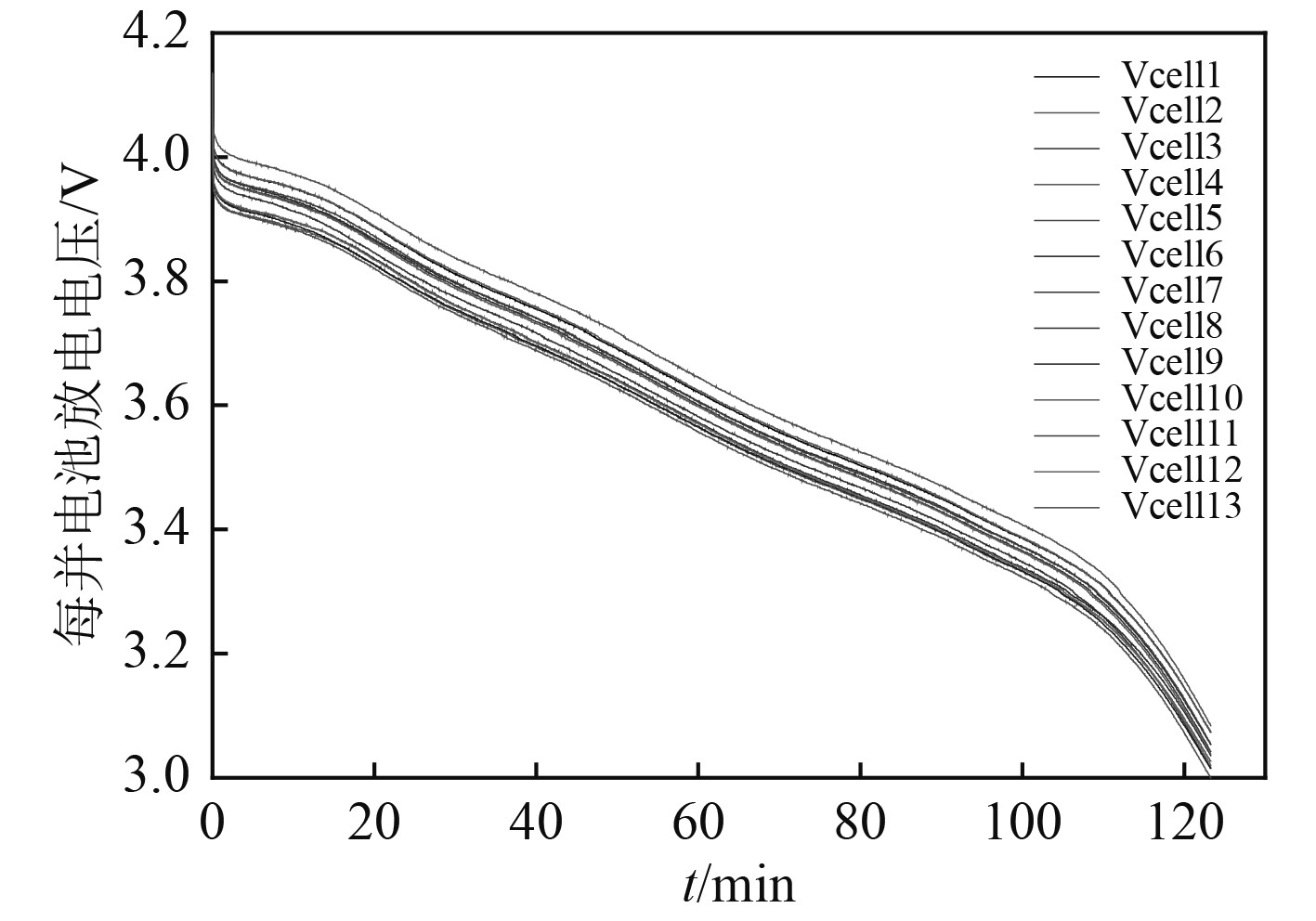图 4 每并电池放电电压曲线 Fig. 4 Discharge voltage curve of each parallel battery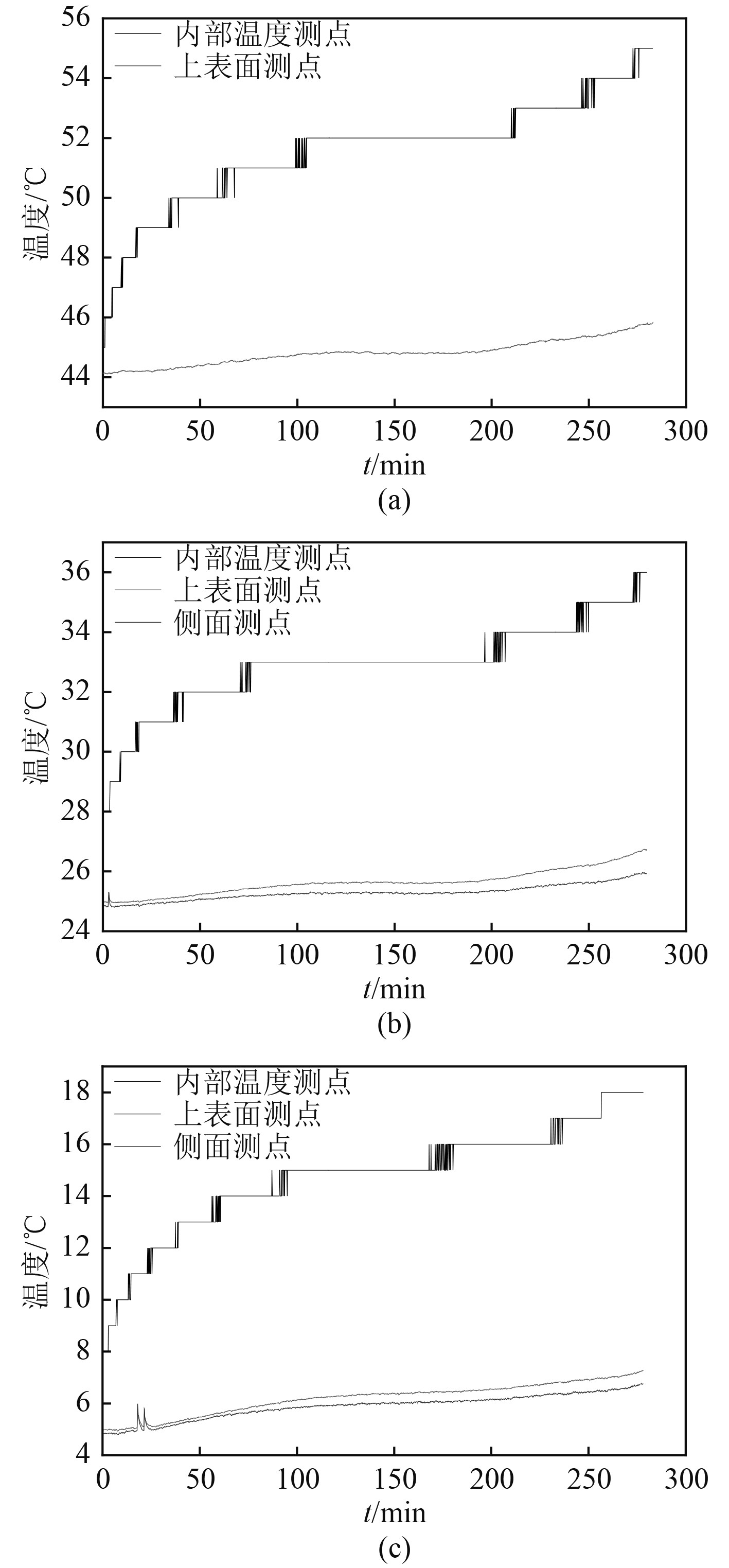图 5 不同环境温度下各温度测点对比 Fig. 5 Comparison of temperature measurement points at different ambient temperatures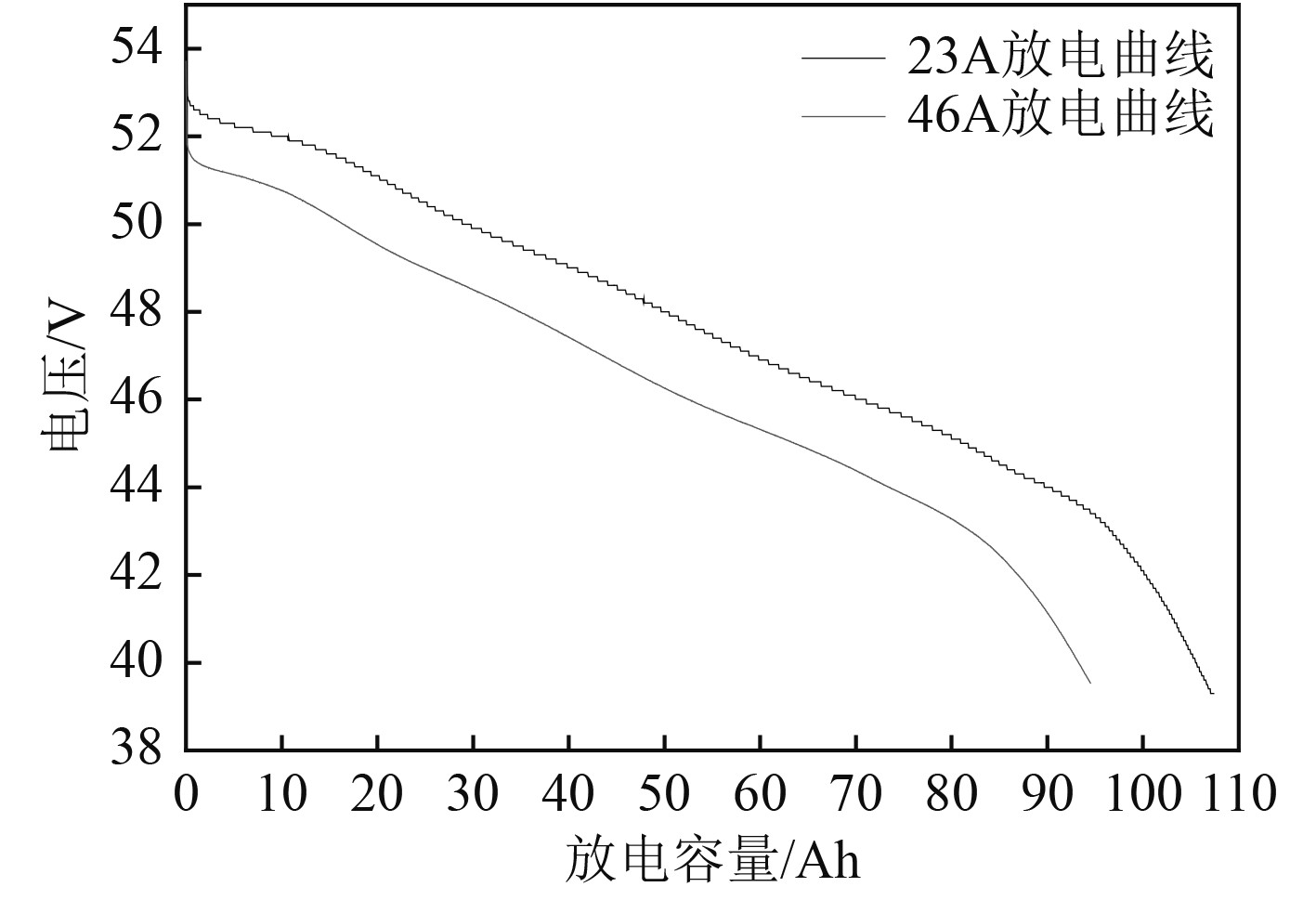图 6 不同放电电流对应放电容量曲线 Fig. 6 The discharge capacity curve corresponding to different discharge currents
4 仿真计算

4.1 网格划分、参数确定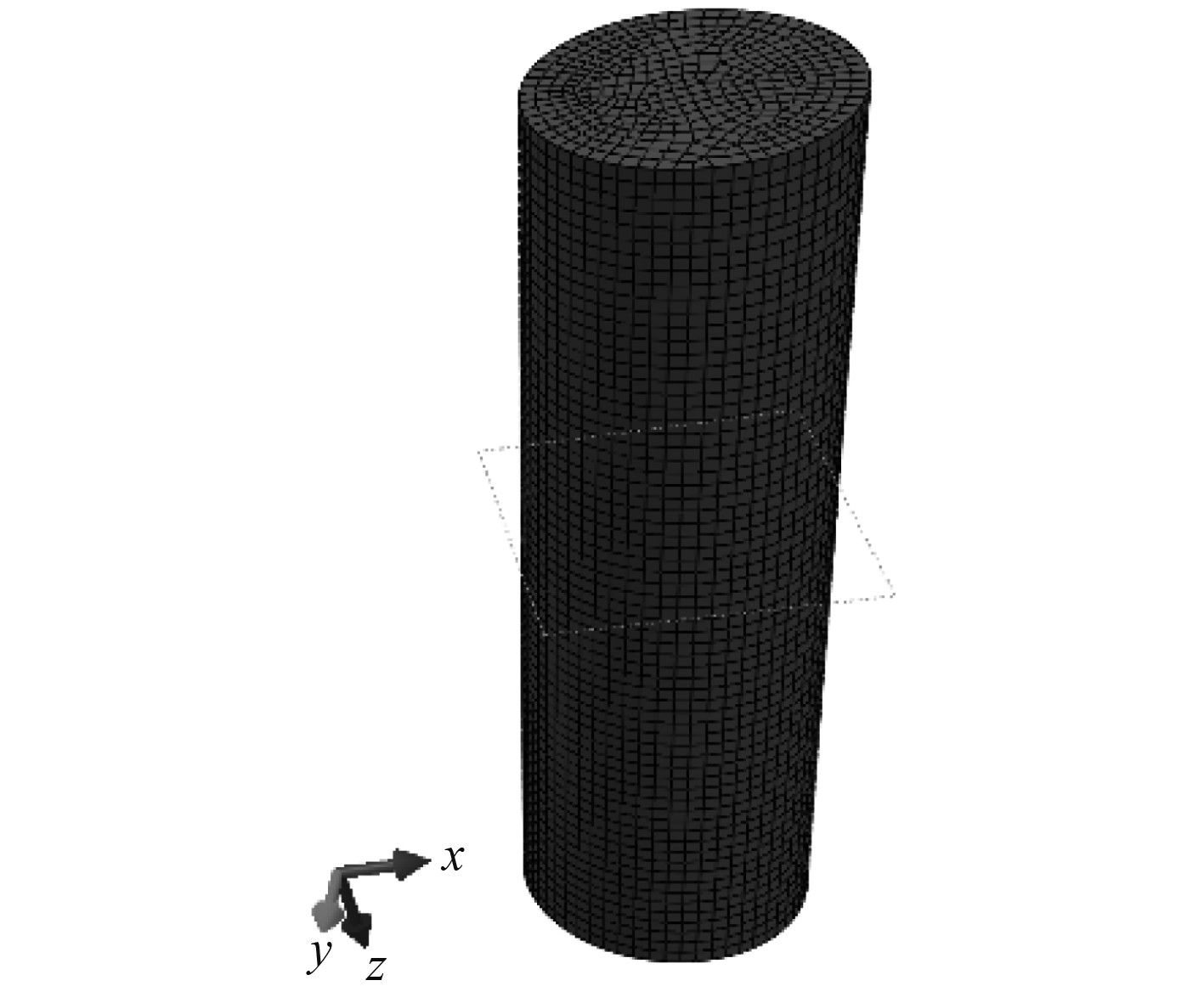图 7 网格划分 Fig. 7 Meshing
4.2 计算结果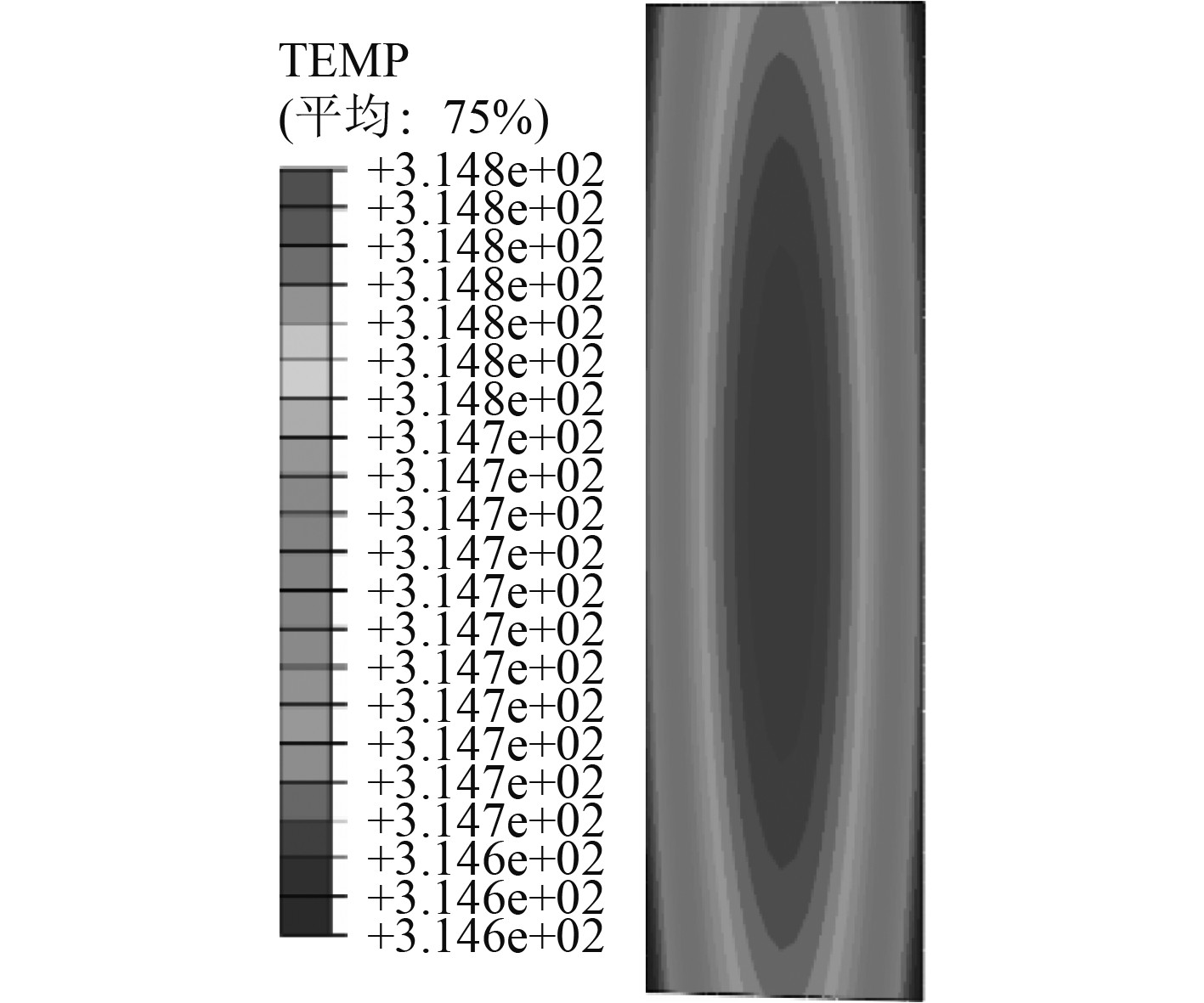图 8 单体电池温度场仿真结果 Fig. 8 Simulation results of temperature field of single cell battery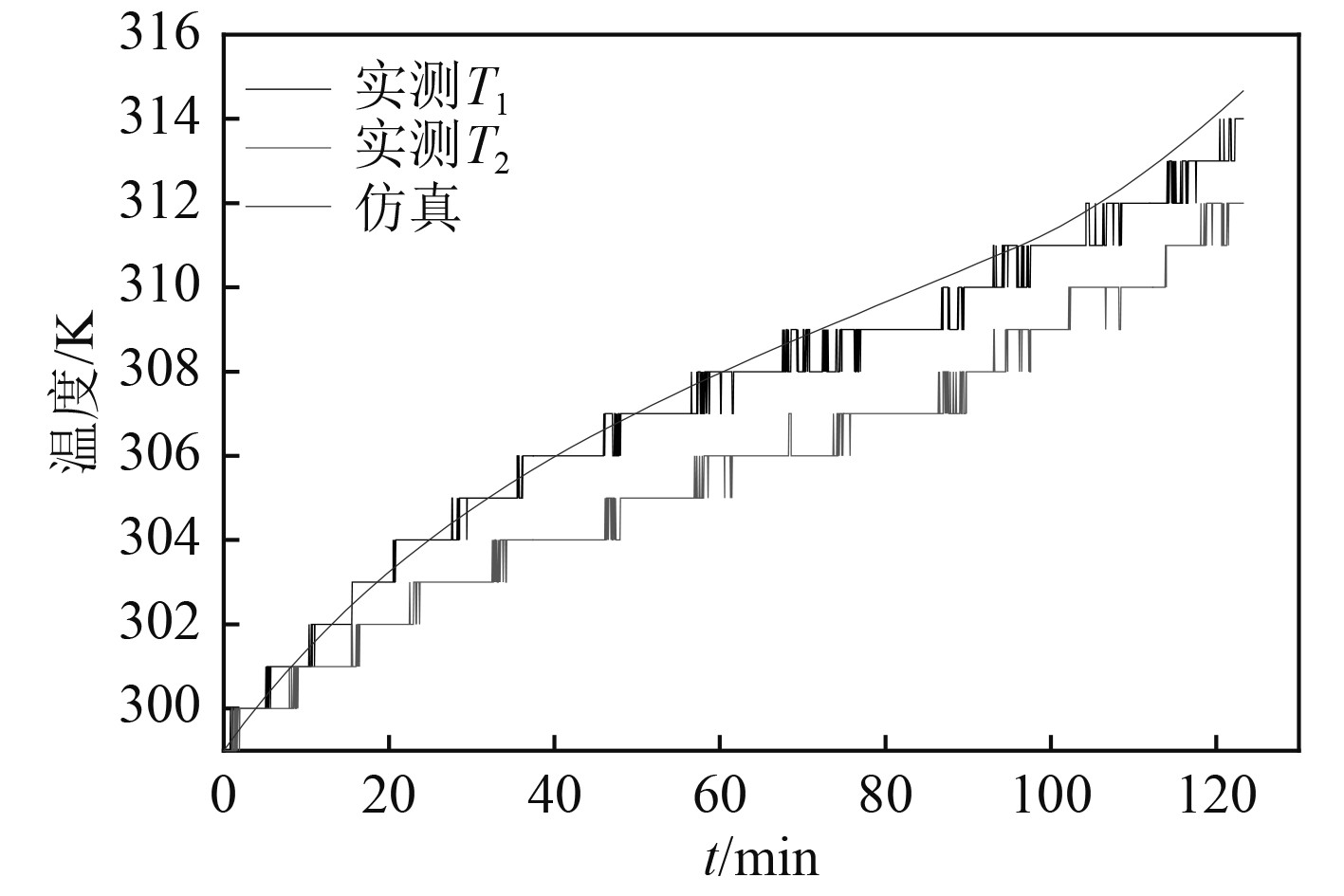图 9 温升特性仿真与试验对比 Fig. 9 Simulation and test comparison of temperature rise characteristic
5 结　语

1）试验结果表明动力电池组在5 ℃～44 ℃使用环境下工作稳定，放电电压、放电容量满足推进器电压需求；

2）单体电池内部温度场呈椭圆分布特征，整体上均匀一致；

3）电池组内部不同位置散热条件差异导致温度场存在不均匀性，但不超过允许最高放电工作温度，实测值与仿真计算结果相近，验证了封闭空间大电流放电时动力电池组的热安全性，可为水下航行器的研制和应用奠定基础。

  陈强. 水下无人航行器[M]. 北京: 国防工业出版社, 2014.  钟宏伟. 国外无人水下航行器装备与技术现状及展望[J]. 水下无人系统学报, 2017(3).  龚锋, 王力. UUV用动力锂电池综述[J]. 船电技术, 2013(8).  CHEN Y F, EVANS J W. Three-dimensional thermal modeling of lithium-polymer batteries under galvanostatic discharge and dynamic power profile[J]. Journal of The Electrochemical Society, 1994, 141(11): 2947-2955. DOI:10.1149/1.2059263  江超. 纯电动汽车用动力电池组热特性研究[D]. 合肥: 合肥工业大学, 2015.